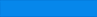# HS III (Comprehensive, focusing on AMC10/12) Fall Semester Syllabus

• Completing Squares
• Circles
• Ellipses
• Hyperbola
• Nonnegative Numbers
• Prime Numbers
• Factors and Divisors
• Arithmetic Sequences
• Geometry Sequences
• System Equations
• Vieta's Theorem
• Matrix

# HS III (Comprehensive, focusing on AMC10/12) Spring Semester Syllabus

• Triangle Areas
• Similar Triangles
• Circles
• Coordinate Geometry
• Angle Bisectors
• Median
• 3D Geometry
• AM-GM Inequality I
• AM-GM Inequality II
• AM-GM Inequality III
• Cauchy's Inequality I
• Cauchy's Inequality II
• Counting by Permutation
• Counting by Geometry